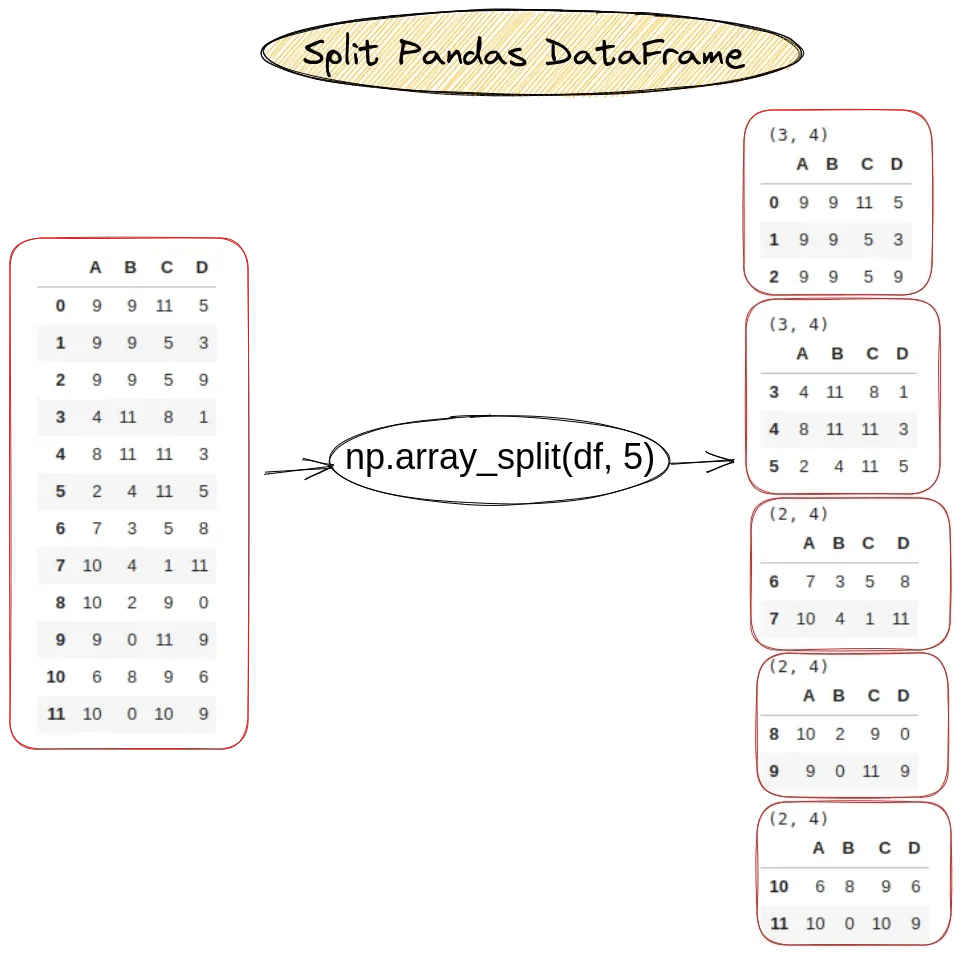In this short guide, I'll show you how to split Pandas DataFrame. You can also find how to:

• split a large Pandas DataFrame
• pandas split dataframe into equal chunks
• split DataFrame by percentage
• split dataset into training and testing parts

To start, here is the syntax to split Pandas Dataframe in 5 equal chunks:

``````import numpy as np
np.array_split(df, 5)
``````

which returns a list of DataFrames. Let's see all the steps in details

## Setup

Lets create a sample DataFrame which contains 12 rows:

``````import pandas as pd
import numpy as np

data = np.random.randint(0,12,size=(12, 4))
df = pd.DataFrame(data, columns=list('ABCD'))
``````

data looks like:

A B C D
0 8 4 7 4
1 1 9 4 5
2 1 4 1 8
3 4 9 3 7
4 8 11 5 9
5 11 9 11 3
6 5 1 6 3
7 2 11 11 7
8 5 9 4 4
9 9 0 11 0
10 4 10 3 8
11 0 0 2 1

## Step 1: Split dataframe into n chunks

Numpy method `np.array_split()` can be used on Pandas DataFrame to split it in n chunks:

``````import pandas as pd
import numpy as np

df = pd.DataFrame(np.random.randint(0,12,size=(12, 4)), columns=list('ABCD'))

chunks = np.array_split(df, 5)

for chunk in chunks:
print(chunk.shape)
display(chunk)
``````

The result is 5 chunks. As you can see the first two chunks has 3 rows while the rest 2 rows:## Step 2: Split DataFrame with list comprehension

To split DataFrame by using list comprehensions we can:

• calculate the chunk size
• get all rows for a given rage
``````chunk_size = df.shape // 3
chunks = [df[i:i+chunk_size].copy() for i in range(0, df.shape, chunk_size)]
``````

This will divide the input DataFrame into 3 DataFrames:

``````[   A  B  C  D
0  8  4  7  4
1  1  9  4  5
2  1  4  1  8
3  4  9  3  7,
A   B   C  D
4   8  11   5  9
5  11   9  11  3
6   5   1   6  3
7   2  11  11  7,
A   B   C  D
8   5   9   4  4
9   9   0  11  0
10  4  10   3  8
11  0   0   2  1]
``````

## Step 3: Split DataFrame into groups

We can use method `groupby` to split DataFrame into:

• equal chunks
• group by criteria

The next code will split DataFrame into equal sized groups:

``````groups = df.groupby(df.index % 4)
``````

Now we can work with each group to get information like count or sum:

``````groups.count()
groups.sum()
``````

result:

A B C D
0 3 3 3 3
1 3 3 3 3
2 2 2 2 2
3 2 2 2 2
4 2 2 2 2

## Step 4: Split DataFrame by column

We can group by a column and then split the DataFrame:

``````groups = df.groupby(df['A'] % 2)
``````

To get information for a group we can use method `first`:

``````groups.first()
``````

or display all groups

``````for group in groups:
display(group)
``````

result:

``````(0,
A   B   C  D
0	8   4   7  4
5   11   9  11  3
10   4  10   3  8)

(1,
A  B  C  D
1   1  9  4  5
6   5  1  6  3
11  0  0  2  1)

(2,
A   B   C  D
2  1   4   1  8
7  2  11  11  7)

(3,
A  B  C  D
3  4  9  3  7
8  5  9  4  4)

(4,
A   B   C  D
4  8  11   5  9
9  9   0  11  0)
``````

## Step 5: Split large DataFrame

To split large DataFrames we can use the Dask library. We can:

• convert the Pandas DataFrame to Dask
• provide number of partitions

To split large DataFrame with Dask we can do:

In case that you need to read huge CSV file and split it to several DataFrames you can use Dask as follow:

``````import dask.dataframe as dd
``````

### Split large DataFrame with Dask

If the DataFrame exists it can be split to chunks by this method `repartition`:

``````df = df.repartition(npartitions=2)
``````

## Step 6: Split dataframe by percentage

Finally let say that you need to split your DataFrame to several parts taking into account percentage. For this purpose we can use `train_test_split` from `sklearn`. We can specify the training and test size as a percentage.

### Split to train and testing data

This is escpecially good for machine learning when you need to split DataFrame into 2 parts for testing and training:

``````from sklearn.model_selection import train_test_split

x, x_test, y, y_test = train_test_split(df,df.index,test_size=0.2,train_size=0.8)
``````

where:

• x - contains the first DataFrame
• y - indexes of first DataFrame
• Index([0, 3, 6, 9, 2, 8, 10, 11, 7], dtype='int64')
• x_test - second DataFrame
• y_test - indexes of the second one
• Index([4, 5, 1], dtype='int64')

### Split by percentage with numpy

We can also use numpy to split the DataFrame into multiple datasets based on percentage from the original data. This can be done by:

``````partitions = [int(.2*len(df)), int(.5*len(df)), int(.6*len(df))]
a, b, c, d = np.split(df, partitions)
``````

So partitions are calculated and defined as:

``````[2, 6, 7]
``````

which selects:

• 2 rows for the first one
• 4 rows for the second
• 1 rows for the 3rd one
• the rest for the 4th

## Conclusion

In this post we saw multiple different ways to split DataFrame into several chunks. We have used different additional packages like `numpy`, `sklearn` and `dask`.

You will know how to easily split DataFrame into training and testing datasets. We also covered how to read a huge CSV file and separate it into multiple DataFrames with Dask.